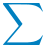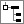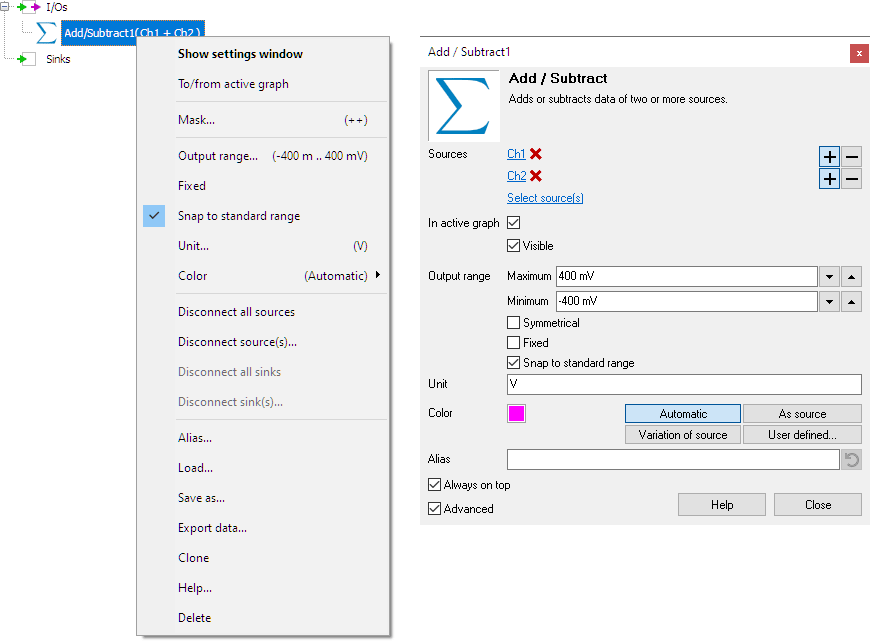The Add / Subtract I/O adds or subtracts data of two or more sources. Up to 32 sources can be added or subtracted with a Add / Subtract I/O.

A typical application of the Add / Subtract I/O is performing a differential measurement using two channels. When the high voltage and the low voltage of the differential signal are measured, the differential signal can be calculated by subtracting the low signal from the high signal.

## Properties

To control the behavior of the Add / Subtract I/O, several properties are available. These can be accessed through a popup menu which is shown when the I/O is right clicked in the Object Tree. The properties can also be accessed through its settings window which is shown when the I/O is double clicked in the Object Tree. To open the Object tree, click theShow object tree button.By default, the settings window only shows the most used settings. When Advanced is ticked, the extended window with all settings is shown. See also the program settings.

The +- mask determines which sources are added and which sources are subtracted. This mask contains a '+' or '-' character for every connected source. By default, the mask consists of '+'-es only and all sources are added. To subtract a source, set its corresponding mask character to '-'.

Some examples of +- masks are shown in the table below:

2 ++ src1 + src2
2 +- src1 - src2
2 -+ src2 - src1
2 -- -src1 - src2
3 +-- src1 - (src2 + src3)
6 +-+--+ (src1 + src3 + src6) - (src2 + src4 + src5)
Hint: To invert a source, you can use an Add / Subtract I/O with a with a mask that is '-'.

### Related information

#### Software generator

The Software generator source (SofGen) generates fully configurable standard signals like sine, block and triangle.

#### Gain / Offset

The Gain / Offset I/O multiplies a signal with a constant gain factor and adds a constant offset.

#### Multiply / Divide

The Multiply / Divide I/O multiplies or divides data of two ore more sources.

#### Sqrt

The SQRT I/O calculates the square root of each sample of the source's data.

#### ABS

The ABS I/O takes the absolute value of each sample of the source's data.

#### Differentiate

The Differentiate I/O differentiates the source's data.

#### Integrate

The Integrate I/O integrates its source's data.

#### Logarithm

The Logarithm I/O calculates the logarithm of the source's data.